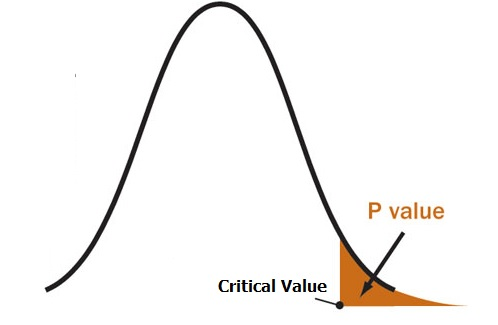# Test Statistic: What is it? Types of Test Statistic

Share on

Statistics Definitions > Test Statistic

You might want to read these articles first:
Reject the Null Hypothesis: What Does it Mean?
What is a P-Value?

A test statistic is used in a hypothesis test when you are deciding to support or reject the null hypothesis. The test statistic takes your data from an experiment or survey and compares your results to the results you would expect from the null hypothesis.

For example, let’s say that you think Drug X will cure genital warts. The currently accepted fact (the null hypothesis) is that about 30% of warts will go away on their own. You conduct a clinical trial and find that 57% of your patients are cured with drug X. Is this a significant result? Does the drug work? Is the 57% a fluke result? You can answer these questions with a test statistic.

## Test Statistics and P-Values

When you run a hypothesis test, you’ll use a distribution like a t-distribution or normal distribution. These have a known area, and enable to you to calculate a probability value (p-value) that will tell you if your results are due to chance, or if your results are die to your theory being correct. The larger the test statistic, the smaller the p-value and the more likely you are to reject the null hypothesis.A p-value is an area in the tail of a distribution that tells you the odds of a result happening by chance.

## Types of Test Statistic

There are four main test statistics you can use in a hypothesis test. Which one you use depends on which statistical test you run. Click on one of the test names or scores to find out more about that particular test.

 Hypothesis Test Test Statistic Z-Test Z-Score T-Test T-Score ANOVA F-statistic Chi-Square Test Chi-square statistic
CITE THIS AS:
Stephanie Glen. "Test Statistic: What is it? Types of Test Statistic" From StatisticsHowTo.com: Elementary Statistics for the rest of us! https://www.statisticshowto.com/test-statistic/
------------------------------------------------------------------------------

Need help with a homework or test question? With Chegg Study, you can get step-by-step solutions to your questions from an expert in the field. Your first 30 minutes with a Chegg tutor is free!